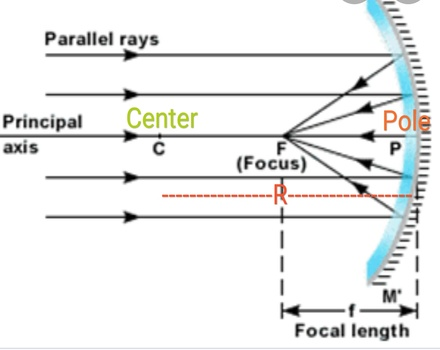# The length of a concave mirror is 10 cm. What will the radius of curvature will be?

## Question:

The length of a concave mirror is 10 cm. What will the radius of curvature will be?

## Concave Mirror:

Concave mirror which is used for converge the light rays after reflection at a point. This point is know as Principal focus.

So , concave mirror is also called converging mirror.

Concave mirror is used in headlights of vehicles.

To find the radius of curvature of a concave mirrorRadius of curvature is defined as the distance from the center of spherical mirror to the pole of mirror.

It is represented by R

Focal length is defined as the distance between the principal focus and the pole of mirror.

It is represented by f.

Relation between the radius of curvature and focal length is

{eq}f = \frac{R}{2} \\ R = 2 f \\ {/eq}

Given

Focal length of a concave mirror (f) = 10 cm

{eq}R = 2 f \\ R = 2\times 10 \\ R = 20 \ cm \\ {/eq}